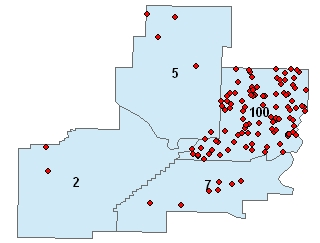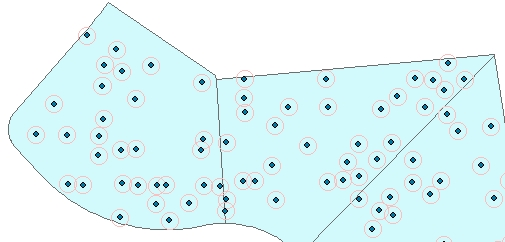## Random Points In Polygons

Running programmatically

Generates random points located in the polygons of the input polygon dataset. The number of points per polygon can be constant or different for each polygon - based on the values in a numeric field of the input polygon feature class.

### Inputs:

• A polygon feature class
• The number of points per polygon can be input in one of the following ways
• A numeric field which values will be used to get the number of points to be generated per polygon.
• A constant number
• Optional: Minimum Distance from boundary - no point will be generated that is closer than this tolerance to the boundary of the polygon.
• Optional: Minimum distance between the points

### Outputs:

• New Point feature class.
• The following fields are added to the attribute table of the resulting feature class.
• [ET_ID] - the ID of the original polygon
• [ET_X] - the X coordinate of the point
• [ET_Y] - the Y coordinate of the point

### Notes:

• If the number of points specified cannot be placed in specific polygon, a message will be stored in the log file.
• The larger minimum distance between the points specified, the more uniform the points created will be.

### Illustration:

Original polygons labeled with the values in field to be used as a source for getting the number of points to be generated and the resulting points. Distance between points = 0, Minimum distance to boundary = 030 points per polygon.
Distance between points = 0, Minimum distance to boundary = 0Distance between points = 0, Minimum distance to boundary = 530 points per polygon. Distance between points = 5, Minimum distance to boundary = 030 points per polygon. Distance between points = 5, Minimum distance to boundary = 5### Running Programmatically

(Go to TOP)

Parameters

Expression Explanation
Function Name RandomPointsInPolygons
<input dataset> A String representing the input layer. Must be of Polygon type.
<output dataset> A String - the full name of the output layer.
{Number Points} An Integer representing the number of points per polygon to be created.
{Number Points Field} A String representing the name of a field in the in the attribute table of the input dataset. The field has the values for the number points per polygon to be created.
{Distance Between} A Double representing the minimum distance between the points to be generated.
{Distance to Boundary} A Double representing the minimum distance to the polygon boundary

### Running the function

ETGWPath used in the table below is the full path to ETGWRun.exe (E.G. "C:\Program Files\ETSpatial Techniques\ETGeo Wizards\ETGWRun.exe")

Language Syntax
Python subprocess.call( [ETGWPath, "RandomPointsInPolygons", "input dataset", "output dataset", "Number Points", "Number Points Field", "Distance Between","Distance to Boundary"])
.NET using ETGWRun.exe StartInfo.FileName = ETGWPath
StartInfo.Arguments = "RandomPointsInPolygons" "input dataset" "output dataset" "Number Points" "Number Points Field" "Distance Between" "Distance to Boundary"
.NET using ETGWOutX.dll RandomPointsInPolygons(input dataset, output dataset, Number Points, Number Points Field, Distance Between, Distance to Boundary)
ArcPy arcpy.RandomPointsInPolygons(input dataset, output dataset, "Number Points", "Number Points Field", "Distance Between", "Distance to Boundary")

Notes:

• <> - required parameter

• {} - optional parameter

• See examples for Python , .NET or ArcPy

• The argument separator for StartInfo.Arguments is space. If a string might contain a space, you need to double quote it.

(Go to TOP)